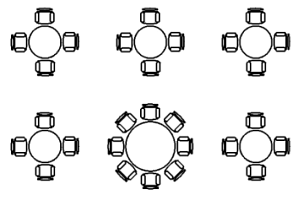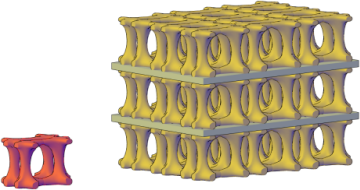# ARRAYRECT (Command)

Distributes object copies into any combination of rows, columns, and levels.

Find2D rectangular array with one item replaced3D rectangular array with three levels

This command is equivalent to the Rectangular option in ARRAY. The DELOBJ system variable controls whether the source objects of the array are deleted or retained after the array is created.

Note: The 3D objects in the example cannot be created in AutoCAD LT.

The following prompts are displayed.

### Select objects

Select the objects to use in the array.

### Associative

Specifies whether the arrayed objects are associative or independent.

Yes
Contains array items in a single array object, similar to a block. With an associative array, you can quickly propagate changes throughout the array by editing the properties and source objects.
No
Creates array items as independent objects. Changes to one item do not affect the other items.

### Base point

Defines the location of the array base point and base point grip.

Base point

Specifies a base point for positioning the items in the array.

Key point

For associative arrays, specifies a valid constraint (or key point) on the source objects to align with the path. If you edit the source objects or path of the resulting array, the base point of the array remains coincident with the key point of the source objects.

### Count

Specifies the number of rows and columns and provides a dynamic view of results as you move the cursor (a quicker alternative to the Rows and Columns options).

Expression

Derives the value based on a mathematical formula or equation.

### Spacing

Specifies row and column spacing and provides a dynamic view of results as you move the cursor.

Distance between rows
Specifies the distance between each row, measured from equivalent locations on each object.
Distance between columns
Specifies the distance between each column, measured from equivalent locations on each object.
Unit cell

Specifies the distance between rows and columns simultaneously by setting the each corner of a rectangular area that is equivalent to the spacing.

### Columns

Edits the number and spacing of columns.

Number of columns

Sets the number of columns in the array.

Distance Between Columns
Specifies the distance between each column, measured from equivalent locations on each object.
Total
Specifies the total distance between the start and end column, measured from equivalent locations on the start and end objects.

### Rows

Specifies the number of rows in the array, the distance between them, and the incremental elevation between row.

Number of rows

Sets the number of rows in the array.

Distance between rows
Specifies the distance between each row, measured from equivalent locations on each object.
Total
Specifies the total distance between the start and end row, measured from equivalent locations on the start and end objects.
Incrementing elevation
Sets the increasing or decreasing elevation for each subsequent row.
Expression

Derives the value based on a mathematical formula or equation.

### Levels

Specifies the number and spacing of levels for 3D arrays.

Number of levels
Specifies the number of levels in the array.
Distance between levels
Specifies the difference in Z coordinate values between equivalent locations on each object.
Total

Specifies the total difference in Z coordinate values between equivalent locations on objects in the first and last levels.

Expression

Derives the value based on a mathematical formula or equation.

### Exit

Exits the command.